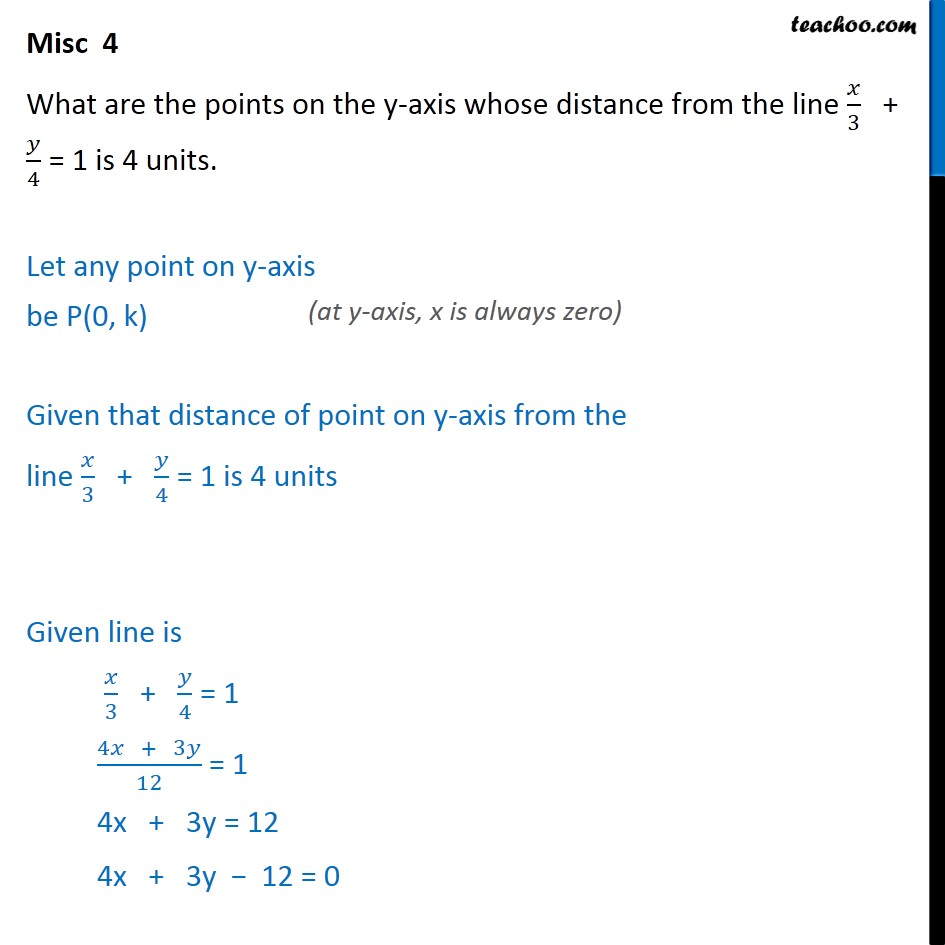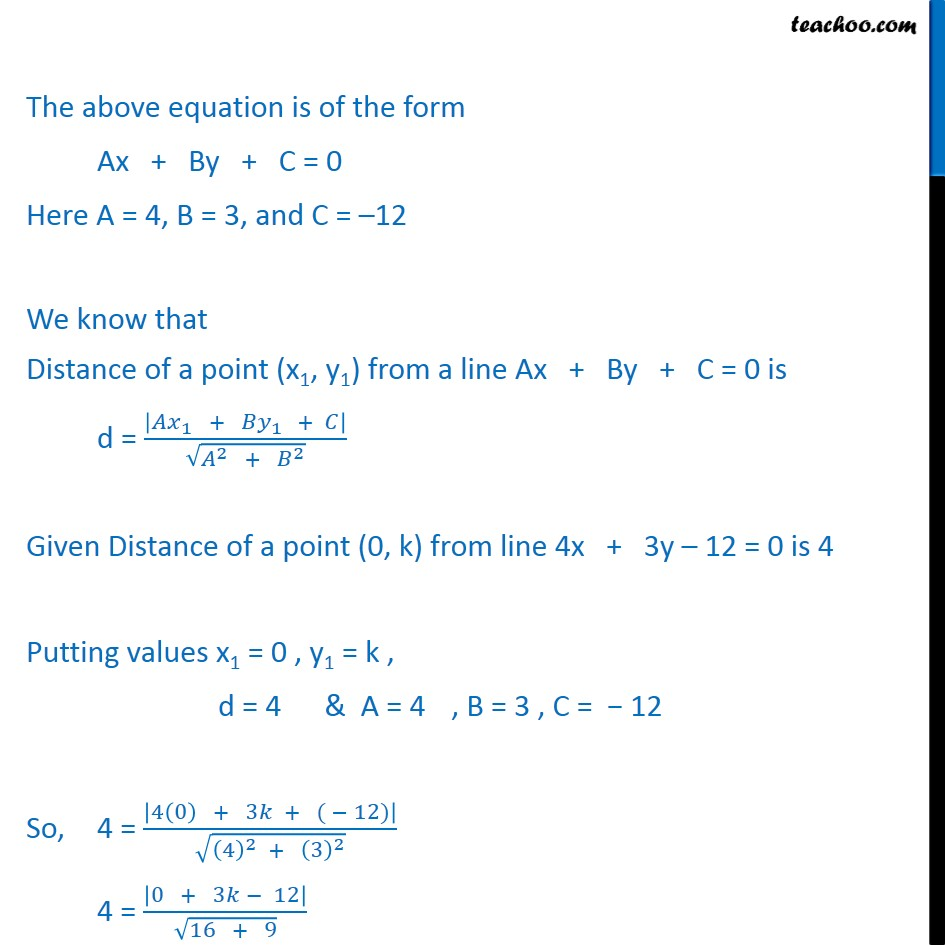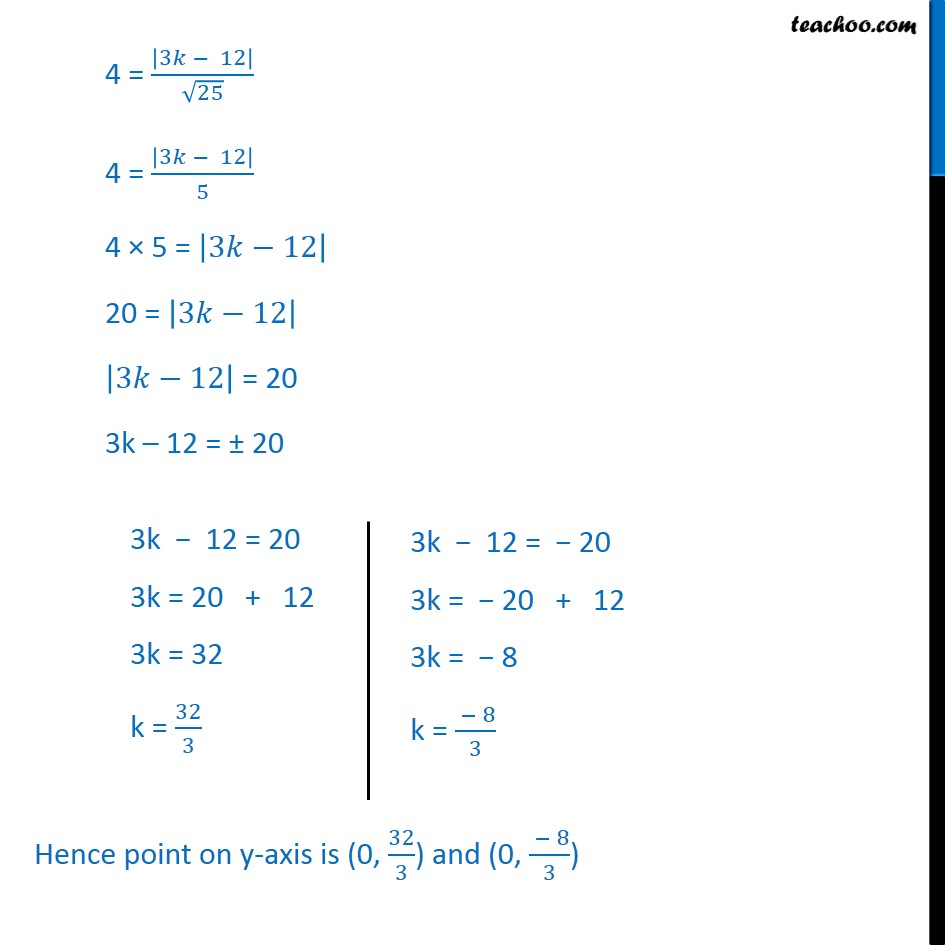Miscellaneous

Chapter 9 Class 11 Straight Lines
Serial order wiseLearn in your speed, with individual attention - Teachoo Maths 1-on-1 Class

### Transcript

Misc 4 What are the points on the y-axis whose distance from the line 𝑥/3 + 𝑦/4 = 1 is 4 units. Let any point on y-axis be P(0, k) Given that distance of point on y-axis from the line 𝑥/3 + 𝑦/4 = 1 is 4 units Given line is 𝑥/3 + 𝑦/4 = 1 (4𝑥 + 3𝑦)/12 = 1 4x + 3y = 12 4x + 3y − 12 = 0 The above equation is of the form Ax + By + C = 0 Here A = 4, B = 3, and C = –12 We know that Distance of a point (x1, y1) from a line Ax + By + C = 0 is d = |〖𝐴𝑥〗_1 + 〖𝐵𝑦〗_1 + 𝐶|/√(𝐴^2 + 𝐵^2 ) Given Distance of a point (0, k) from line 4x + 3y – 12 = 0 is 4 Putting values x1 = 0 , y1 = k , d = 4 & A = 4 , B = 3 , C = − 12 So, 4 = |4(0) + 3𝑘 + ( − 12)|/√((4)^2 + (3)^2 ) 4 = |0 + 3𝑘 − 12|/√(16 + 9) 4 = |3𝑘 − 12|/√25 4 = |3𝑘 − 12|/5 4 × 5 = |3𝑘−12| 20 = |3𝑘−12| |3𝑘−12| = 20 3k – 12 = ± 20 Hence point on y-axis is (0, 32/3) and (0, ( − 8)/3)